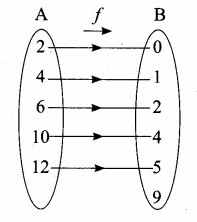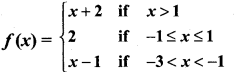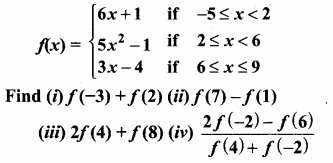# Chapter 1 Relations and Functions, Ex 1.4, Tamilnadu board, sslc,

22 Nov 2020 7:09 pm

### Question 2.

Let f: A → B be a function defined by
f(x) = x2 – 1, where A = {2, 4,6,10,12},
B = {0,1,2,4,5,9}. Represent f by
(i) set of ordered pairs
(ii) a table
(iii) an arrow diagram
(iv) a graph
Answer:
A = {2,4,6, 10, 12}
B = {0,1, 2, 4, 5, 9}
f(x) = x2 – 1
f(2) = 22 – 1 = 1 – 1 = 0
f(4) = 42 – 1 = 2 – 1 = 1
f(6) = 62 – 1 = 3 – 1 = 2
f(10) = 102 – 1 = 5 – 1 = 4
f(12) = 122 – 1 = 6 – 1 = 5

(i) Set of ordered pairs
f = {(2, 0) (4, 1) (6, 2) (10, 4) (12, 5}

(ii) Table

(iii) Arrow diagram(iv) Graph

Question 3.
Represent the function f = {(1,2), (2,2), (3,2), (4,3),(5,4)} through (i) an arrow diagram (it) a table form (iii) a graph.
Answer:
f = {(1, 2) (2, 2) (3, 2) (4, 3) (5,4)}
Let A = {1,2, 3, 4, 5}
B = {2, 3, 4}

(i) Arrow diagram

(ii) Table form

(iii) Graph

Question 4.
Show that the function f : N → N defined by f(x) = 2x – 1 is one-one but not onto.
Answer:
f: N → N
N = {1,2,3,4,5,… }
f(x) = 2x – 1
f(1) = 2(1) – 1 = 2 – 1 = 1
f(2) = 2(2) – 1 = 4 – 1 = 3
f(3) = 2(3) – 1 = 6 – 1 = 5
f(4) = 2(4) – 1 = 8 – 1 = 7
f(5) = 2(5) – 1 = 10 – 1 = 9
f = {(1,1) (2, 3) (3, 5) (4, 7) (5,9) …..}

(i) Different elements has different images. This function is one to one function.
(ii) Here Range is not equal to co-domain. This function not an onto function.
∴ The given function is one-one but not an onto.

Question 5.
Show that the function f: N ⇒ N defined by f(m) = m2 + m + 3 is one-one function.
Answer:
N = {1,2,3, 4,5, ….. }
f(m) = m2 + m + 3
f(1) = 12 + 1 + 3 = 5
f(2) = 22 + 2 + 3 = 9
f(3) = 3+ 3 + 3 = 15
f(4) = 42 + 4 + 3 = 23
f = {(1,5) (2, 9) (3, 15) (4, 23)}

From the diagram we can understand different elements in (N) in the domain, there are different images in (N) co-domain.
∴ The function is a one-one function.

Question 6.
Let A = {1, 2, 3, 4) and B = N. Letf: A → B be
defined by f(x) = x3 then,
(i) find the range off
(ii) identify the tpe of function

Solution:
A = {1, 2, 3, 4}
B = N
f: A → B,f(x) = x3
(i) f(1) = 13 = 1
f(2) = 23 = 8
f(3) = 33 = 27
f(4) = 43 = 64
(ii) Therange of f = {1, 8, 27, 64 )
(iii) It is one-one and into function.

Question 7.
In each of the following cases state whether the function is bijective or not. Justify your answer.
(i) f: R → R defined by f (x) = 2x + 1
(ii) f: R → R defined by f(x) = 3 – 4x2

Answer:
(i) f(x) = 2x + 1
f(0) = 2(0) + 1 = 0 + 1 = 1
f(1) = 2(1) + 1 = 2 + 1 = 3
f(2) = 2(2) + 1 = 4 + 1 = 5
f(3) = 2(3) + 1 = 6 + 1 = 7
Different elements has different images
∴ It is an one-one function.
It is also an onto function. The function is one-one and onto function.
∴ It is a bijective function.

(ii) f(x) = 3 – 4x2
f(1) = 3 – 4(1)2
= 3 – 4 = -1
f(2) = 3 – 4(2)2 = 3 – 16 = – 13
f(3) = 3 – 4(3)2 = 3 – 36 = – 33
f(4) = 3 – 4(42) = 3 – 64 = – 61
It is not a bijective function. The positive numbers “R” do not have negative pre – image in X in R.

Question 8.
Let A= {-1,1}and B = {0,2}.
If the function f: A → B defined by
f(x) = ax + b is an onto function?

Find a and b.

Answer:
A = {-1, 1}; B = {0,2}
f(x) = ax + b
f(-1) = a(-1) + b
0 = -a + b
a – b = 0 ….(1)
f(1) = a(1) + b
2 = a + b
a + b = 2 ….(2)
Solving (1) and (2) we get

Substitute a = 1 in (1)
The value of a = 1 and b = 1

Question 9.
If the function f is defined byfind the value of
(i) f(3)
(ii) f(0)
(iii) f(1. 5)
(iv) f(2) + f(-2)
Answer:
f(x) = x + 2 when x = {2,3,4,……}
f(x) = 2
f(x) = x – 1 when x = {-2}
(i) f(x) = x + 2
f(3) = 3 + 2 = 5

(ii) f(x) = 2
f(0) = 2

(iii) f(x) = x – 1
f(-1.5) = -1.5 – 1 = -2.5

(iv) f(x) = x + 2
f(2) = 2 + 2 = 4
f(x) = x – 1
f(-2) = – 2 – 1 = – 3
f(2) + f(-2) = 4 – 3
= 1

Question 10.
A function f: [-5, 9] → R is defined as follows:Answer:
f(x) = 6x + 1 ; x = {-5,-4,-3,-2,-1,0,1}
f(x) = 5x2 – 1 ; x = {2, 3, 4, 5}
f(x) = 3x – 4 ; x = {6, 7, 8, 9}

(i) f(-3) + f(2)
f(x) = 6x + 1
f(-3) = 6(-3) + 1 = -18 + 1 = -17
f(x) = 5x2 – 1
f(2) = 5(2)2 – 1 = 20 – 1 = + 19
f(-3) + f(2) = – 17 + 19
= 2

(ii) f(7) – f(1)
f(x) = 3x – 4
f(7) = 3(7) – 4 = 21 – 4 = 17
f(x) = 6x + 1
f(1) = 6(1) + 1 = 6 + 1 = 7
f(7) – f(1) = 17 – 7
= 10

(iii) 2f(4) + f(8)
f(x) = 5x2 – 1
f(4) = 5(4)2 – 1 = 5(16) – 1
= 80 – 1 = 79
f(x) = 3x – 4
f(8) = 3(8) – 4 = 24 – 4 = 20
2f(4) + f(8) = 2(79) + 20
= 158 + 20
= 178f(x) = 6x + 1
f(-2) = 6(-2) + 1 = -12 + 1 = -11
f(x) = 3x – 4
f(6) = 3(6) – 4 = 18 – 4 = 14
f(x) = 5x2 – 1
f(4) = 5(4)2 – 1 = 5(16) – 1
= 80 – 1 = 79
f(x) = 6x + 1
f(-2) = 6(-2) + 1 = -12 + 1 = -11

Question 11.
The distance S an object travels under the influence of gravity in time t seconds is given by S(t) = 12 gt2 + at + b where, (g is the acceleration due to gravity), a, b are constants. Check if the function S (t)is one-one.

Solution:

S(t) = 12 gt2 + at + b
Let t be 1, 2, 3, ………, seconds
S(1) = 12 g(12) + a(1) + b = 12 g + a + b
S(2) = 12 g(22) + a(2) + b = 2g + 2a + b
Yes, for every different values of t, there will be different values as images. And there will be different preimages for the different values of the range. Therefore it is one-one function.

Question 12.
The function ‘t’ which maps temperature in Celsius (C) into temperature in Fahrenheit (F) is defined by
t(C) = F where F = 95 C + 32. Find,
(i) t(0)
(ii) t(28)
(iii) t(-10)
(iv) the value of C when t(C) = 212
(v) the temperature when the Celsius value is equal to the Fahrenheit value.

Answer:

Given t(C) = 9C5 + 32

(i) t(0) = 9(0)5 + 32
= 32° F

(ii) t(28) = 9(28)5 + 32
= 2525 + 32
= 50.4 + 32
= 82.4° F

(iii) t(-10) = 9(−10)5 + 32
= -18 + 32
= 14° F

(iv) t(C) = 212
9C5 + 32 = 212
9C5 = 212 – 32
= 180
9C = 180 × 5
C = 180×59
= 100° C

(v) consider the value of C be “x”
t(C) = 9C5 + 32
x = 9×5 + 32
5x = 9x + 160
-160 = 9x – 5x
-160 = 4x
x = −1604 = -40
The temperature when the Celsius value is equal to the fahrenheit value is -40°

Composition of two Functions

Let f: A → B and g: B → C be two functions. Then the composition of f and g denoted by gof is defined as the function gof (x) = g[f(x)] for all x ∈ A.

Composition of three Functions

Let A, B, C, D be four sets and let f: A → B; g : B → C and h : C → D be three functions, using composite functions fog and goh, we get two new functions like (fog) oh and fo (goh).

Note: Composition of three function is always associative.

#### VISITORS COUNTUsers Today : 512Total Users : 87826Views Today : 1827Total views : 365146